# RS Aggarwal Class 7 Solutions Chapter 5 Exponents CCE Test Paper

## RS Aggarwal Class 7 Solutions Chapter 5 Exponents CCE Test Paper

These Solutions are part of RS Aggarwal Solutions Class 7. Here we have given RS Aggarwal Solutions Class 7 Chapter 5 Exponents CCE Test Paper.

Other Exercises

Question 1.
Solution: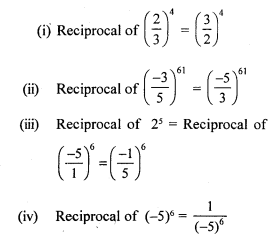Question 2.
Solution:
Let the required number be x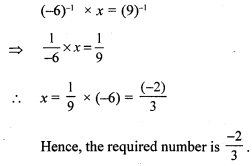Question 3.
Solution:
Let the required number be x.
(-20)-1 ÷ x = (-10)-1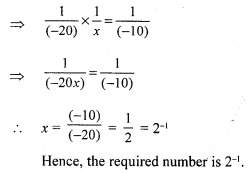Question 4.
Solution:
(i) 2000000 = 2.000000 x 106 [Since the decimal point is moved 6 to the left]
= 2 x 106
(ii) 6.4 x 105 = 6.4 x 100000 = 640000

Question 5.
Solution:
We have :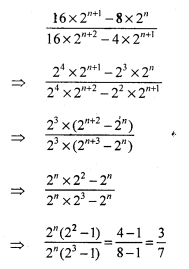Question 6.
Solution:
We have :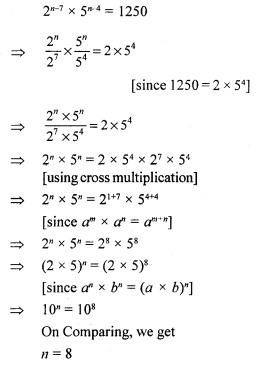Mark (✓) against the correct answer in each of the following :
Question 7.
Solution: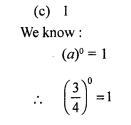Question 8.
Solution: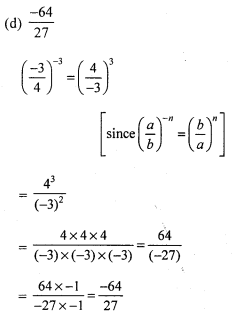Question 9.
Solution: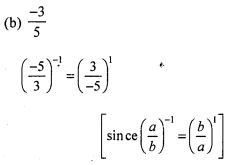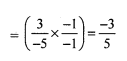Question 10.
Solution: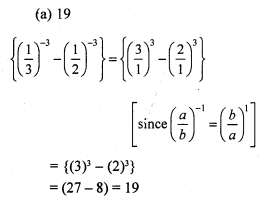Question 11.
Solution: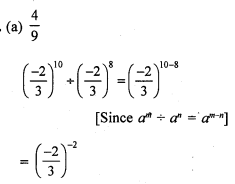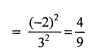Question 12.
Solution:
(c) 3.263 x 105
A given number is said to be in standard form if it can be expressed as
k x 10n, where k is a real number such that 1 < k < 10 and n is a positive integer.
For example : 3.263 x 105

Question 13.
Solution: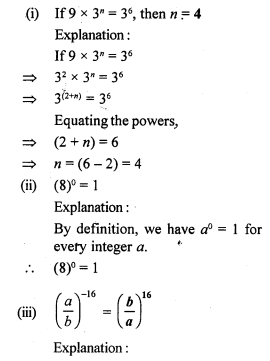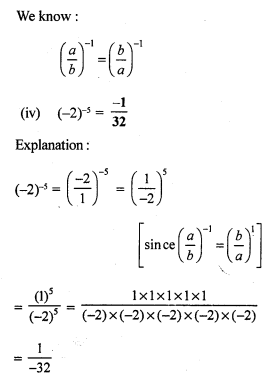Question 14.
Solution:
(i) True
645 = 6.45 x 102
[Since the decimal point is moved 2 places to the left]
(ii) False
27000 = 2.7 x 104
[Since the decimal point is moved 4 places to the left]
(iii) False
(3° + 4° + 5°) = 1
(iv) False
Reciprocal of 56 = Reciprocal of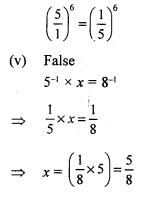Hope given RS Aggarwal Solutions Class 7 Chapter 5 Exponents CCE Test Paper are helpful to complete your math homework.

If you have any doubts, please comment below. Learn Insta try to provide online math tutoring for you.